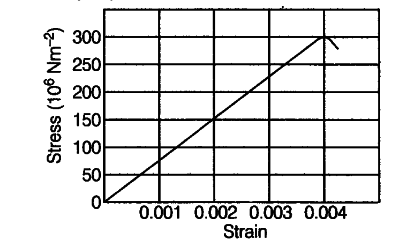# What are Young's modulus

Fig. shows the strain-stress curve for a given material. What are (i) Young’s modulus and (ii) approximate yield strength far this material?(i) Youngs modulus of the given material (Y)
= Slope of strain-stress curve
Y = 150 x\${{10}^{6}}\$/ 0.002=75x\${{10}^{9}}\$ N/\${{m}^{2}}\$

= 7.5x \${{10}^{10}}\$ N/\${{m}^{2}}\$
(ii) Yield strength of the given material
= Maximum stress the material can sustain

= 300 X\${{10}^{6}}\$ N/\${{m}^{2}}\$ = 3 X\${{10}^{8}}\$ N/\${{m}^{2}}\$